# NEET Physics Systems of Particles and Rotational Motion Questions Solved

A block of mass m moving at a velocity v collides with another block of mass 2m at rest. The lighter block comes to rest after collision. Find the coefficient of restitution.

(a) $\frac{1}{2}$                                               (b) $1$

(c) $\frac{1}{3}$                                               (d) $\frac{1}{4}$

Concept Videos :-

#4 | Definition of Momentum
#5 | Conservation of Momentum
#6 | Problems

Concept Questions :-

Linear momentum
Complete Question Bank + Test Series
Complete Question Bank

Difficulty Level:

A small mass attached to a string rotates on a frictionless table top as shown. If the tension on the string is increased by pulling the string causing the radius of the circular motion to decrease by a factor of 2, the kinetic energy of the mass will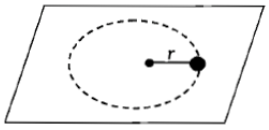(a) Increase by a factor of 4                                       (b) Decrease by a factor of 2

(c) Remain constant                                                   (d) Increase by a factor of 2

Concept Videos :-

#19 | Angular Momentum

Concept Questions :-

Angular momentum
Complete Question Bank + Test Series
Complete Question Bank

Difficulty Level: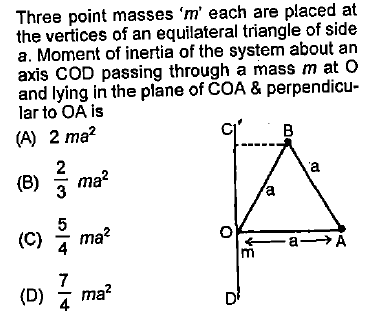Concept Videos :-

#10 | Moment of Inertia (MI): Introduction
#11 | MI: Discreet Particles
#12 | MI: Continuous Bodies_1
#13 | MI: Questions on Continuous Bodies
#14 | MI: Continuous Bodies_2
#15 | Theorems of Moment of Inertia
#16 | Questions on Theorems of MI
#17 | Set 1: Previous Year Problems

Concept Questions :-

Moment of inertia
Complete Question Bank + Test Series
Complete Question Bank

Difficulty Level: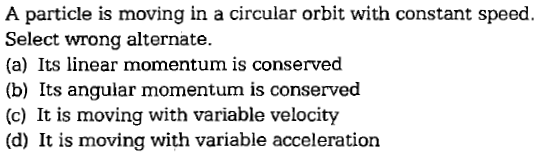Concept Videos :-

#4 | Definition of Momentum
#5 | Conservation of Momentum
#6 | Problems

Concept Questions :-

Linear momentum
Complete Question Bank + Test Series
Complete Question Bank

Difficulty Level: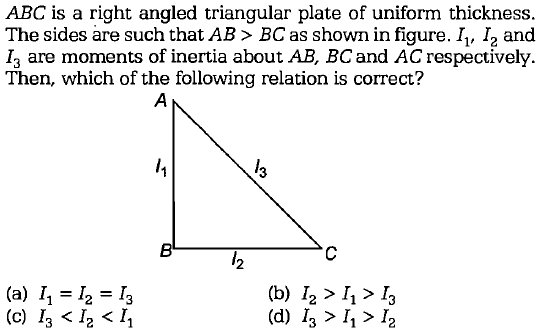Concept Videos :-

#10 | Moment of Inertia (MI): Introduction
#11 | MI: Discreet Particles
#12 | MI: Continuous Bodies_1
#13 | MI: Questions on Continuous Bodies
#14 | MI: Continuous Bodies_2
#15 | Theorems of Moment of Inertia
#16 | Questions on Theorems of MI
#17 | Set 1: Previous Year Problems

Concept Questions :-

Moment of inertia
Complete Question Bank + Test Series
Complete Question Bank

Difficulty Level: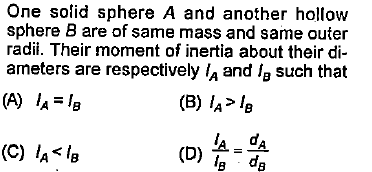Concept Videos :-

#10 | Moment of Inertia (MI): Introduction
#11 | MI: Discreet Particles
#12 | MI: Continuous Bodies_1
#13 | MI: Questions on Continuous Bodies
#14 | MI: Continuous Bodies_2
#15 | Theorems of Moment of Inertia
#16 | Questions on Theorems of MI
#17 | Set 1: Previous Year Problems

Concept Questions :-

Moment of inertia
Complete Question Bank + Test Series
Complete Question Bank

Difficulty Level:

A couple produces [NTSE 1995; CBSE PMT 1997; DCE 2004]

(1) Purely linear motion

(2) Purely rotational motion

(3) Linear and rotational motion

(4) No motion

Complete Question Bank + Test Series
Complete Question Bank

Difficulty Level:

A particle of mass 1 kg is kept at (1m, 1m, 1m). The moment of inertia of this particle about z-axis would be

1.

2.

3.

4.  None of these

Complete Question Bank + Test Series
Complete Question Bank

Difficulty Level:

One quarter sector is cut from a uniform circular disc of radius R.  This sector has mass M.  It is made to rotate about a line perpendicular to its plane and passing through the centre of the original disc.  Its moment of inertia about the axis of rotation is [IIT-JEE (Screening) 2001]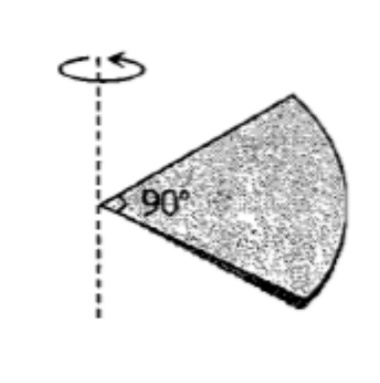(1) $\frac{1}{2}M{R}^{2}$

(2) $\frac{1}{4}M{R}^{2}$

(3) $\frac{1}{8}M{R}^{2}$

(4) $\sqrt{2}M{R}^{2}$

Complete Question Bank + Test Series
Complete Question Bank

Difficulty Level:

A wheel is rotating at the rate of 33 rev/min.  If it comes to stop in 20 s. Then, the angular retardation will be

(1) $\mathrm{\pi }\frac{\mathrm{rad}}{{\mathrm{s}}^{2}}$                          (2)

(3)               (4)